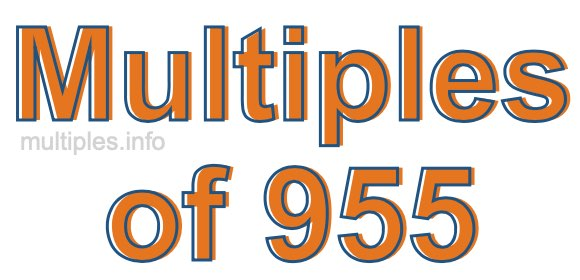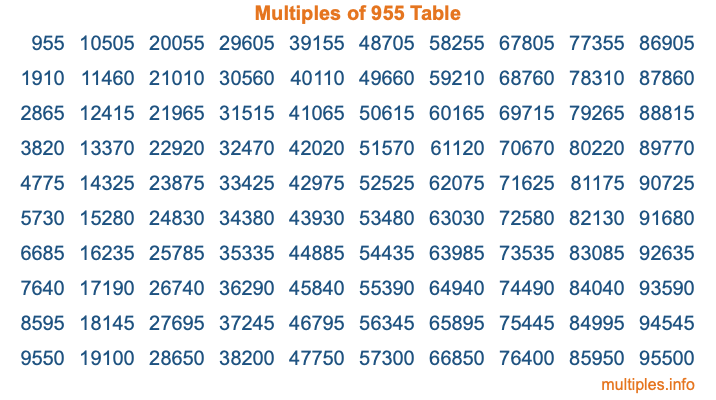Multiples of 955Welcome to the Multiples of 955 page. Here we will first teach you everything you will ever need to know about the multiples of 955, and then give you a study guide summary of everything we taught you to make sure you remember it all. Use this page to look up facts and learn information about the multiples of 955. This page will make you a multiples of nine hundred fifty-five expert!

Definition of Multiples of 955
Multiples of 955 are all the numbers that when divided by 955 equal an integer. Each of the multiples of 955 are called a multiple. A multiple of 955 is created by multiplying 955 by an integer.

Therefore, to create a list of multiples of 955, you start with 1 multiplied by 955, then 2 multiplied by 955, then 3 multiplied by 955, and so on for as long as you want. Thus, the list of the first five multiples of 955 is 955, 1910, 2865, 3820, and 4775. To see a larger list of multiples of 955, see the printable image of Multiples of 955 further down on this page. We also have a category where you can choose any nth multiple of 955.

Multiples of 955 Checker
The Multiples of 955 Checker below checks to see if any number of your choice is a multiple of 955. In other words, it checks to see if there is any number (integer) that when multiplied by 955 will equal your number. To do that, we divide your number by 955. If the the quotient is an integer, then your number is a multiple of 955.

Is  a multiple of 955?

Least Common Multiple of 955 and ...
A Least Common Multiple (LCM) is the lowest multiple that two or more numbers have in common. This is also called the smallest common multiple or lowest common multiple and is useful to know when you are adding our subtracting fractions. Enter one or more numbers below (955 is already entered) to find the LCM.

Check out our LCM Calculator if you need more details about the Least Common Multiple or if you need the LCM for different numbers for adding and subtraction fractions.

nth Multiple of 955
As we stated above, 955 is the first multiple of 955, 1910 is the second multiple of 955, 2865 is the third multiple of 955, and so on. Enter a number below to find the nth multiple of 955.

th multiple of 955

Multiples of 955 vs Factors of 955
955 is a multiple of 955 and a factor of 955, but that is where the similarities end. All postive multiples of 955 are 955 or greater than 955. All positive factors of 955 are 955 or less than 955.

Below is the beginning list of multiples of 955 and the factors of 955 so you can compare:

Multiples of 955: 955, 1910, 2865, 3820, 4775, etc.

Factors of 955: 1, 5, 191, 955

As you can see, the multiples of 955 are all the numbers that you can divide by 955 to get a whole number. The factors of 955, on the other hand, are all the whole numbers that you can multiply by another whole number to get 955.

It's also interesting to note that if a number (x) is a factor of 955, then 955 will also be a multiple of that number (x).

Multiples of 955 vs Divisors of 955
The divisors of 955 are all the integers that 955 can be divided by evenly. Below is a list of the divisors of 955.

Divisors of 955: 1, 5, 191, 955

The interesting thing to note here is that if you take any multiple of 955 and divide it by a divisor of 955, you will see that the quotient is an integer.

Multiples of 955 Table
Below is an image of the first 100 multiples of 955 in a table. The table is in chronological order, column by column. The first column has the first ten multiples of 955, the second column has the next ten multiples of 955, and so on.The Multiples of 955 Table is also referred to as the 955 Times Table or Times Table of 955. You are welcome to print out our table for your studies.

Negative Multiples of 955
Although not often discussed or needed in math, it is worth mentioning that you can make a list of negative multiples of 955 by multiplying 955 by -1, then by -2, then by -3, and so on, to get the following list of negative multiples of 955:

-955, -1910, -2865, -3820, -4775, etc.

Multiples of 955 Summary
Below is a summary of important Multiples of 955 facts that we have discussed on this page. To retain the knowledge on this page, we recommend that you read through the summary and explain to yourself or a study partner why they hold true.

There are an infinite number of multiples of 955.

A multiple of 955 divided by 955 will equal a whole number.

955 divided by a factor of 955 equals a divisor of 955.

The nth multiple of 955 is n times 955.

The largest factor of 955 is equal to the first positive multiple of 955.

955 is a multiple of every factor of 955.

955 is a multiple of 955.

A multiple of 955 divided by a divisor of 955 equals an integer.

955 divided by a divisor of 955 equals a factor of 955.

Any integer times 955 will equal a multiple of 955.

Multiples of a Number
Here you can get the multiples of another number, all with the same attention to detail as we did for multiples of 955 on this page.

Multiples of
Multiples of 956
Did you find our page about multiples of nine hundred fifty-five educational? Do you want more knowledge? Check out the multiples of the next number on our list!